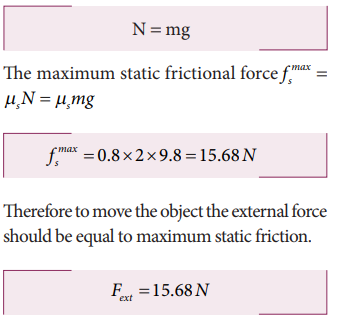Home | | Physics 11th std | Solved Example Problems for Friction

# Solved Example Problems for Friction

Physics : Laws of Motion : Friction

## Solved Example Problems for Static Friction

### Example 3.17

Consider an object of mass 2 kg resting on the floor. The coefficient of static friction between the object and the floor is µ s = 0.8. What force must be applied on the object to move it?

### Solution

Since the object is at rest, the gravitational force experienced by an object is balanced by normal force exerted by floor.Therefore to move the object the external force should be equal to maximum static friction.

### Example 3.18

Consider an object of mass 50 kg at rest on the floor. A Force of 5 N is applied on the object but it does not move. What is the frictional force that acts on the object?

### Solution

When the object is at rest, the external force and the static frictional force are equal and opposite.

The magnitudes of these two forces are equal, f s = Fext

Therefore, the static frictional force acting on the object is

Fs=5N

The direction of this frictional force is opposite to the direction of Fext .

### Example 3.19

Two bodies of masses 7 kg and 5 kg are connected by a light string passing over a smooth pulley at the edge of the table as shown in the figure. The coefficient of static friction between the surfaces (body and table) is 0.9. Will the mass m1 = 7 kg on the surface move? If not what value of m2 should be used so that mass 7 kg begins to slide on the table?

### Solution

As shown in the figure, there are four forces acting on the mass m1

a) Downward gravitational force along the negative y-axis (m1g)

b) Upward normal force along the positive y axis (N)

c) Tension force due to mass m2 along the positive x axis

d) Frictional force along the negative x axis

Since the mass m2 has no vertical motion, m1= NTo determine whether the mass m1 moves on the surface, calculate the maximum static friction exerted by the table on the mass m1. If the tension on the mass m1 is equal to or greater than this maximum static friction, the object will move.The tension T = m2 g = 5X9.8 = 49 NThe tension acting on the mass m1 is less than the maximum static friction. So the mass m1 will not move.

To move the mass m1T = fsmax where T = m2gIf the mass m2 is 6.3 kg then the mass m1 will begin to slide. Note that if there is no friction on the surface, the mass m1 will move for m2even for just 1 kg.

The values of coefficient of static friction for pairs of materials are presented in Table 3.1. Note that the ice and ice pair have very low coefficient of static friction. This means a block of ice can move easily over another block of ice.

## Solved Example Problems for Angle of Repose

### Example 3.20

A block of mass m slides down the plane inclined at an angle 60° with an acceleration g/2. Find the coefficient of kinetic friction?

### Solution

Kinetic friction comes to play as the block is moving on the surface.

The forces acting on the mass are the normal force perpendicular to surface, downward gravitational force and kinetic friction fk along the surface.There is no motion along the y-direction as normal force is exactly balanced by the mg cosθ .## Solved Example Problems for Friction

### Example 3.21

Consider an object moving on a horizontal surface with a constant velocity. Some external force is applied on the object to keep the object moving with a constant velocity. What is the net force acting on the object?### Solution

If an object moves with constant velocity, then it has no acceleration. According to Newton’s second law there is no net force acting on the object. The external force is balanced by the kinetic friction.

Study Material, Lecturing Notes, Assignment, Reference, Wiki description explanation, brief detail
11th Physics : UNIT 3 : Laws of Motion : Solved Example Problems for Friction |